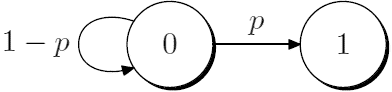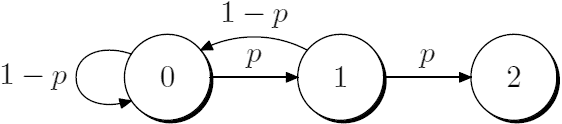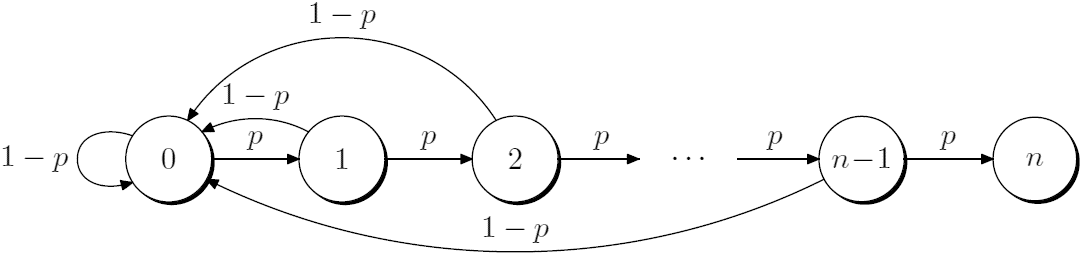## 平均投几个三分才会连续进 3 个？

2012年8月3日 发表评论 阅读评论$N_1=p\cdot 1 + (1-p)(1+N_1)$.

$\displaystyle N_1=\frac{1}{p}.$$N_2=p^2\cdot 2 + p(1-p)(2+N_2) + (1-p)(1+N_2)$

$\displaystyle N_2=\frac{1+p}{p^2}.$$N_3=p^3\cdot 3 + p^2(1-p)(3+N_3) + p(1-p)(2+N_3) + (1-p)(1+N_3)$

$\displaystyle N_3=\frac{1+p+p^2}{p^3}.$$N_n=p\cdot (N_{n-1}+1) + (1-p)(N_{n-1}+1+N_n)$

$\displaystyle N_n=\frac{N_{n-1}+1}{p}.$

$\displaystyle N_n=\frac{1+p+p^2+\cdots+p^{n-1}}{p^n}.$

$\displaystyle N_n=\frac{1-p^n}{p^n(1-p)}=\frac{p^{-n}-1}{1-p}.$

1. 本文目前尚无任何评论.
1. 2013年7月1日18:04 | #1